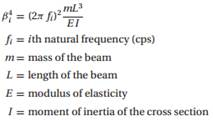Create an Account

Home / Questions / The natural frequencies of a uniform cantilever beam are related to the roots βi of the fr...

The natural frequencies of a uniform cantilever beam are related to the roots βi of the frequency equation

The natural frequencies of a uniform cantilever beam are related to the roots βi of the frequency equation f(β) = cosh β cos β + 1 = 0, whereDetermine the lowest two frequencies of a steel beam 0.9 m long, with a rectangular cross section 25 mm wide and 2.5 mm in. high. The mass density of steel is 7850 kg/m3 and E = 200 GPa.

Jul 27 2020 View more View LessSubscribe To Get Solution